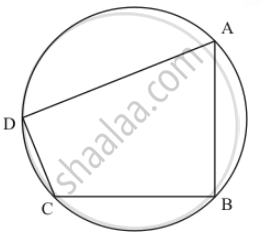Advertisement Remove all ads

# In a cyclic quadrilateral ABCD, if m ∠A = 3 (m ∠C). Find m ∠A. - Mathematics

Short Note

In a cyclic quadrilateral ABCD, if A = 3 (m ∠C). Find m ∠A.

Advertisement Remove all ads

#### Solution

It is given that

ABCD is cyclic quadrilateral and    m angle A = 3 (m  angle C )We have to find  m angle A

Since ABCD is cyclic quadrilateral and sum of opposite pair of cyclic quadrilateral is 180°.

So  angle A + angle C = 180°

And

3angleC + angleC = 180°

4angleC = 180°

angleC = (180°)/4

= 45°

Therefore

angleA = 3 xx 45°

= 135°

Hence  angle A = 135°

Is there an error in this question or solution?
Advertisement Remove all ads

#### APPEARS IN

RD Sharma Mathematics for Class 9
Chapter 15 Circles
Exercise 15.5 | Q 10 | Page 101
Advertisement Remove all ads
Advertisement Remove all ads
Share
Notifications

View all notifications

Forgot password?
Course